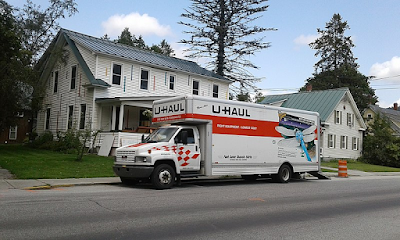## Tuesday, September 28, 2021

### Another Rook Tour on the Chessboard

In  the following problem is described:

1. The chessboard shown above has $$8\times 8 = 64$$ cells.
2. Place numbers 1 through 64 in the cells such that the next number is either directly left, right, below, or above the current number.
3. The dark cells must be occupied by prime numbers. Note there are 18 dark cells, and 18 primes in the sequence $$1,\dots,64$$. These prime numbers are: 2,3,5,7,11,13,17,19,23,29,31,37,41,43,47,53,59,61.

This problem looks very much like the Numbrix puzzle . We don't have the given cells that are provided in Numbrix, but instead, we have some cells that need to be covered by prime numbers.

The path formed by the consecutive values looks like how a rook moves on a chessboard. Which explains the title of this post (and of the original post). If we require a proper tour we need values 1 and 64 to be neighbors. Otherwise, if this is not required, I think a better term is: Hamiltonian path. I'll discuss both cases, starting with the Hamiltonian path problem.

### A covering problem: runners vs Ragnar relay legs

In  the following problem was posted.

1. We have a number of legs with their lengths (in miles). E.g.

legs = [3.3, 4.2, 5.2, 3, 2.7, 4,
5.3, 4.5, 3, 5.8, 3.3, 4.9,
3.1, 3.2, 4, 3.5, 4.9, 2.3,
3.2, 4.6, 4.5, 4, 5.3, 5.9,
2.8, 1.9, 2.1, 3, 2.5, 5.6,
1.3, 4.6, 1.5, 1.2, 4.1, 8.1]

2. We also have a number of runs performed by runners. Again, we have distances in miles:

runs = [3.2, 12.3, 5.2, 2.9, 2.9, 5.5]

A run can be allocated to different legs, as long a the sum of these legs is less than the length of the run.
3. We want to maximize the number of legs covered by the runs, but with a wrinkle: the legs should be consecutive: 1,2,3,... (we can't skip a leg).
4. A solution for this data set can look like:

----     60 PARAMETER report  results

run1        run2        run3        run4        run5        run6

leg1                       3.3
leg2                       4.2
leg3                                   5.2
leg4           3.0
leg5                                               2.7
leg6                       4.0
leg7                                                                       5.3
total          3.0        11.5         5.2         2.7                     5.3
runLen         3.2        12.3         5.2         2.9         2.9         5.5


It is noted that solutions are not necessarily unique. In this case, we could have swapped run 4 for run 5 to cover leg 5.

## Friday, September 17, 2021

### Reallocate people: a very small but interesting LPU-Haul Moving Van [link]

This is a strange little problem . We have individuals (of different categories) and regions with a given capacity. The initial set of assigned persons fits within the capacity of each region. Now a new batch of individuals arrives with their chosen zones. Unfortunately, the total demand (old + new individuals) does not fit anymore in all cases. This means we need to reallocate people. We can do this at a price (the costs are given). What is the optimal (least cost) new allocation?

## Friday, September 10, 2021

### Huber regression: different formulationsPeter Huber, Swiss statistician

Traditionally, regression, well-known and widely used, is based on a least-squares objective: we have quadratic costs. A disadvantage of this approach is that large deviations (say due to outliers) get a very large penalty. As a result, these outliers can affect the results dramatically.

One alternative regression method that tries to remedy this is L1- or LAD-regression (LAD=Least Absolute Deviation) . Here I want to discuss a method that is somewhere in the middle between L1 regression and least-squares: Huber or M-Regression .  Huber regression uses a loss function that is quadratic for small deviations and linear for large ones. It is defined in a piecewise fashion. The regression can look like the following non-linear optimization problem:

NLP Model
\begin{align} \min_{\color{darkred}\beta,\color{darkred}\varepsilon} &\sum_i \rho(\color{darkred}\varepsilon_i) \\ &\color{darkblue}y_i = \sum_j \color{darkblue}x_{i,j}\color{darkred}\beta_j +\color{darkred}\varepsilon_i \end{align} where $\rho(\color{darkred}\varepsilon_i) = \begin{cases}\color{darkred}\varepsilon^2_i & \text{if |\color{darkred}\varepsilon_i | \le \color{darkblue}k} \\ 2\color{darkblue}k |\color{darkred}\varepsilon_i |-\color{darkblue}k^2 & \text{otherwise}\end{cases}$